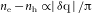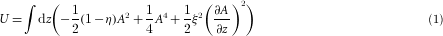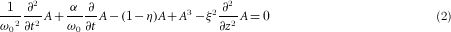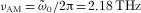## Main

The behaviour of symmetry breaking phase transitions (SBTs) is commonly studied under near-equilibrium (near-ergodic) conditions, where excitations on all timescales contribute to the process, and the behaviour of physical quantities through the SBT is described by power laws with critical exponents. However, when the ordering proceeds non-ergodically, the quasiparticles and collective boson excitations perceive the crystal background as an effective vacuum. To study this behaviour in condensed-matter systems, we chose crystals with electronically driven instabilities caused by a Fermi-surface nesting, leading to a second-order transition to a charge-density-wave (CDW) ordered state7,8,9,10,11,12. The state is characterized by spatial modulations cos(Q x+φ) of the electronic density and lattice displacements described by a complex order parameter ΨΔeiφ. The elementary bosonic collective excitations of Δ and φ are the amplitude and the phase modes (AM and PhM), and Ψ may be viewed as a ‘Higgs field’1, opening a gap 2|Δ| in the fermionic spectrum. In this paper we focus on TbTe3 (CDW transition at Tc=336 K; refs 13, 14), but experiments on other microscopically diverse systems (DyTe3, 2H-TaSe2 and K0.3MoO3) are also presented, demonstrating universality.

The main idea realized here is to quench the system into the high-symmetry state with intense ‘destruction’ (D) laser pulses and then monitor the time evolution through the SBT by measuring the resulting reflectivity oscillations with a pump–probe (P–p) sequence (Fig. 1a). The D pulse excites electrons and holes, thus suppressing the electronic susceptibility at 2kF, whose divergence is the cause for the CDW formation. Any asymmetry in the band structure also leads to an imbalance of the e and h populations, shifting the chemical potential and causing a disturbance δ q of the Fermi surface kF according toand destroying the CDW. After initial rapid quasiparticle (QP) relaxation, we can expect the appearance of topologically non-trivial local configurations—domain walls, solitons and so on, which are allowed by the ground-state degeneracy with respect to φ (ref. 15). (The experimental details are given in the Supplementary Information).Figure 1: Schematic representation of the experiment and the time-resolved response at different times after the destruction of the ordered state.

The transient reflectivity ΔR/R of TbTe3 as a function of D–P time delay Δt12 is shown in Fig. 1b. We distinctly observe an exponential QP transient at short times Δt23<1 ps, and an oscillatory response owing to AM and coherent phonon oscillations14. A two-dimensional plot highlighting the QP response is shown in Fig. 2a. Immediately after the quench the QP peak amplitude AQP is completely suppressed, indicating the disappearance of the CDW gap. As the QP peak starts to recover, initially the QP lifetime τQP is a few picoseconds, but both AQP and τQP recover to their equilibrium values within 1–2 ps. A single-exponential fit to both τQP(t) and AQP(t) is shown in Fig. 2b, giving the QP gap recovery time ττQP =τAQP =650±50 fs. This behaviour is consistent with the previously reported relation τQP1/Δ(T) (refs 14, 16).Figure 2: Evolution of the quasiparticle response and collective mode after the destruction pulse.

Figure 1c shows ΔR/R with the QP signal subtracted, showing an aperiodic oscillatory response for short Δt12. The fast Fourier transform power spectra of these data as a function of Δt12 are plotted in Fig. 2c. The most obvious non-trivial observation in the υ–Δt12 plots is that the intensity of the AM fluctuates strongly up to 7 ps. The fluctuations are irregular at first, showing a distinct slowing down in the critical region Δt12=1.5 ps. The AM, whose frequency in the equilibrium broken-symmetry state is 2.18 THz, shows a dramatic softening for Δt12<2 ps. In the course of the system recovery, the AM crosses the 1.75 THz phonon mode, and a Fano interference effect is clearly observed around t121 ps, similar to the one observed in the temperature dependence14. Significantly, the spectra seem strongly distorted around Δt12=3.5–4 ps, showing asymmetric diagonal ‘blobs’. After 6 ps the fluctuations die down and the AM intensity eventually reaches full amplitude in approximately 60 ps.

To model the evolution of the system through the SBT, we describe Ψ using a weakly dispersive Ginzburg–Landau model. Ignoring phase fluctuations (phase perturbations can be ignored on short timescales, because they require significant time for nucleation), the potential energy of the system can be described by a double-well, rather than a ‘Mexican hat’, potential:Its time dependence is shown schematically in Fig. 3a. Here A(t,z)=Δ(t,z)/Δeq is the time-space-dependent amplitude of Ψ, normalized to the equilibrium value Δeq, and ξ is the coherence length. The function 1−η(t,z) is a parameter describing the perturbation, akin to the temperature deviation (TTc) from criticality in usual Ginzburg–Landau theory. For spatially uniform A(t), η(t)=η(0)exp(−t/τAQP ) as shown in Fig. 3b, where τAQP is determined from fits to Fig. 2b. The time evolution of U and μ=1−η with η(0)=2 is shown schematically in Fig. 3. Before the D pulse, and for large t or z, η=0, the system is ordered and |A|=1. After the D pulse, 1−η<0 and the double-well potential disappears in favour of a single energy minimum at A=0. As 1−η increases and becomes positive, two minima emerge at ±Amin=±(1−η)1/2, and start to attract the system, breaking the symmetry. From equation (1), the equation of motion can be written asHere ω0 is the angular frequency of the bare (2kF) phonon mode responsible for the CDW formation. The second term describes its damping α≤ΔνAM/νAM. The exponentially decaying light intensity due to the finite penetration depth of light is accounted for by the excitation function η′(t,z)=η(t)exp(−z/λ), where λ=20 nm is the light absorption depth of TbTe3 at 800 nm. Using the experimental values for τQP,, linewidth ΔνAM=0.2 THz and coherence length ξ=1.2 nm (ref. 9), we can compute A(t,z). In Fig. 3b we first plot the spatially homogeneous solution with ξ=0, with and without the P pulse. The final ground state is ergodically uncorrelated with the initial one, so the formation of domains is expected under inhomogeneous conditions.Figure 3: The system is modelled by a Ginzburg–Landau model with a time-dependent potential. The oscillatory solutions to the model can be directly compared with experiments.

The full inhomogeneous solution A(t,z) to equation (2) is plotted in Fig. 4a. We see that after 1 ps four domains are formed parallel to the surface, with A(t,z) oscillating either around 1 or −1 (orange or blue respectively), accompanied by the emission of propagating A(t,z)-field waves. At 3 ps we observe the fusion of two domain walls, which is accompanied by the emission of field waves of A(t,z) propagating towards the surface and into the bulk (arrows). They seem to reach the surface around Δt12 4–5 ps, which—as we shall see—causes detectable distortions of the spectra at around 5–6 ps. (More A-field wave dynamics is shown in the accompanying movies.)Figure 4: The predicted evolution of the domain structure and the behaviour of the collective mode through the quench.

In Fig. 4b we show the predicted fast Fourier transform power spectra taking full account of spatial inhomogeneity for the D, P and p pulses. (The response function is derived in the Supplementary Information). The main features of our data in Fig. 2c are unmistakably present: oscillations of A(t) at short times and the critical slowing of the AM oscillations close to the critical point tc1.5 ps. The calculation also reproduces the softening of the AM for Δt12<2 ps. After 2 ps, the ripples in A(t,z) discussed above cause a temporal deformation of the spectral profiles, giving diagonal blobs at 56 ps shown in Fig. 4b. These are remarkably similar to the diagonal spectral distortions observed in the experimental data in Fig. 2c. Exhaustive modelling presented in the Supplementary Information unambiguously shows that the diagonal distortions in νt12 plots are caused by the annihilation of defects.

Further experiments on other microscopically diverse systems (2H-TaSe2, K0.3MoO3 and DyTe3) presented in the Supplementary Information show that the sequence of events after the quench, ultrafast QP gap recovery →Ψ-field amplitude fluctuations → critical slowing down through ts and domain creation → coherent defect annihilation, is commonly observed in the tellurides and the selenide. The microscopic properties of the underlying vacuum such as λ and ξ may change the details, so K0.3MoO3 does not show step (4), which we attribute to departure from universality16. Note that the mechanism described here for topological defect creation is conceptually and historically related not only to vortex formation in superconductivity, but also to the Kibble–Zurek mechanism for the formation of cosmic strings. The Ψ(t,z) waves such as we observe after annihilation events have a direct analogue in the Higgs spontaneous symmetry breaking mechanism. All these models share a common underlying potential, albeit with different microscopic properties of the underlying vacuum and different symmetries of order parameter 1,2,3,5,17. A notable distinction of our system is that φ relaxation is slow compared with the relaxation of the potential itself, enabling the collective mode and topological defect dynamics to be uniquely observed.